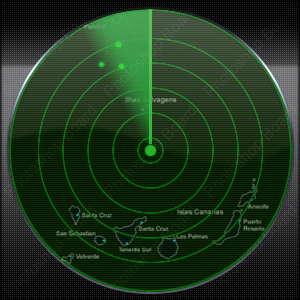Rare
0/16

# Sweep Line

Authors: Benjamin Qi, Andi Qu, Dustin Miao, Timothy Gao

Introduction to line sweep.

### Prerequisites

Imagine you have a vertical line that "sweeps" the plane from left to right. That's the main idea behind the sweep line.

You might be thinking "wait - isn't keeping track of the sweep line at all possible positions super inefficient?" And you'd be correct. However, we don't actually need to keep track of the sweep line at all possible positions - only at the "critical" positions (e.g. points and intersections).

### This section is not complete.

Any help would be appreciated! Just submit a Pull Request on Github.

should the reader already be familiar with the 1D case (union of intervals on number line?) never explicitly mentioned in module

## Closest Pair

Focus Problem – try your best to solve this problem before continuing!

### Solution 1

Time Complexity: $\mathcal{O}(n\log n)$

We will use a divide and conquer algorithm. First, sort the points by x-coordinate. Now, let $P$ be the subarray of points in the current step. Then, partition $P$ into two groups $L$ and $R$ representing the left and right halves of $P$. Let $\delta_l$ and $\delta_r$ be the answer of $L$ and $R$ respectively, and define $\delta$ as $\min(\delta_l, \delta_r)$.

Then $\delta$ is the upperbound of the answer. If a more optimal answer exists, it must bridge the two halves of the array (i.e. one of its endpoints is in $L$ and the other is in $R$). Let $mx$ be the x-coordinate of any median of $P$. Define two sets $L'$ and $R'$ such that $L' = \{p_i | x_i \leq mx, mx - x_i \leq \delta\}$ and $R' = \{p_i | x_i \geq mx, x_i - mx \leq \delta\}$

A brute force matching algorithm that computes $\texttt{dist}(p, q)$ for all $p \in L'$, $q \in R'$ would have a worst-case runtime of $(\frac n 2)^2 = \mathcal{O}(n^2)$ (recall that $L'$ and $R'$ may have up to $\frac N 2$ points). However, because we are searching for distances of at most $\delta$, it suffices for each $p \in L'$ to check all points $\{q | q \in R', p.y - \delta \leq q.y \leq p.y + \delta\}$.

It can be shown that for each point $p$, there is a constant number of points that satisfy this property. Because each point in $R'$ is at least $\delta_r \geq \delta$ apart, arranging the points in the worst case would result in 6 points in the corners and sides of the bounding rectangle.

To achieve the desired $\mathcal{O}(n)$ complexity per layer, we need to be able to efficiently get the points sorted by both x-coordinate (for dividing $P$) and y-coordinate (for matching between $L'$ and $R'$). This can be achieved by taking advantage of the merge-sort-like algorithm: sort by x-coordinate in the beginning, then for each step, merge the y-coordinates recursively.

Because each step now runs in linear time and there is a total of $\lceil \log n \rceil$ steps, by the master theorem our solution now runs in $\mathcal{O}(n\log n)$.

### Solution 2

Time Complexity: $\mathcal{O}(n\log n)$

Extending Solution 1, we can use a set instead of divide and conquer. Once again, we define $\delta$ as the shortest distance between two points so far. After sorting the points by x-coordinate, we iterate through them while maintaining a running window containing the y-coordinates of all points in $[x-\delta,x]$.

As we visit point $P$, we utilize the set to consider all points with y-coordiate in $[P_y-\delta, P_y+\delta]$. The set contains $[P_x-\delta, P_x]$ because of how it is maintained as a running window. Now we have the same bounding box as Solution 1, with at most 6 points inside.

For each point, we recalculate $\delta = min(\delta, \delta_p)$, and update our set accordingly. Each point is inserted and removed from the set at most once, so the algorithm yields $\mathcal{O}(n\log n)$.

## Line Segments

Focus Problem – try your best to solve this problem before continuing!

### This section is not complete.

Any help would be appreciated! Just submit a Pull Request on Github.

### Problems

StatusSourceProblem NameDifficultyTags
Old SilverEasy
Show TagsSweep Line
Old GoldNormal
Show TagsSweep Line
COIHard
Show TagsSweep Line
CEOIHard
Show TagsSweep Line
CEOIVery Hard
Show TagsSweep Line
Baltic OIVery Hard
Show TagsSweep Line

## Manhattan MST

Focus Problem – try your best to solve this problem before continuing!

(KACTL code)

explanation? topcoder prob has

StatusSourceProblem NameDifficultyTags
CSAHard
Show TagsManhattan MST

Instead of a vertical line sweeping the plane from left to right, radial sweep involves a ray that rotates around a central point (like a radar screen):In this case, we sort points/events by their bearing instead of by their x- and y-coordinates. Besides that, the mechanics are the same as those of normal line sweep.

Focus Problem – try your best to solve this problem before continuing!

### Solution - Seeing the Boundary

Complexity: $\mathcal O(N + Rp_i \log R)$

In this problem, there are three types of events: when our ray hits a fence post, enters a rock, or exits a rock.

The second and third types of events can be found for each rock by sorting the rays to its vertices by bearing and then taking the two endpoints of the sorted list. These two rays are the two tangents to the rock.

We can then perform a radial sweep to find the fence posts that Farmer Don can see - these fence posts are simply the ones where the number of type-2 and type-3 events we've processed so far are equal.

Note that some optimizations (e.g. not constructing the list of fence posts explicitly) may be required to get 100 points.

C++

#include <bits/stdc++.h>#define x first#define y secondtypedef long long ll;using namespace std;
const double PI = 4 * atan(1);
struct Event {	short type, id;

### Problems

StatusSourceProblem NameDifficultyTags
CEOIEasy
Show TagsBinary Search, Radial Sweep
CSESEasy
Show Tags1DRQ, Sweep Line
POINormal